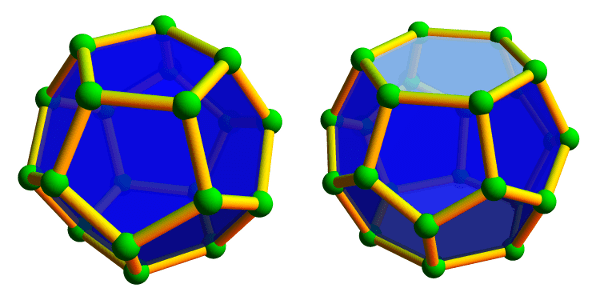# Bucky’s Dozen

Buckyballs are remarkable structures, and not just to mathematicians. In chemistry, a buckyball—more correctly, a spherical fullerene—is a molecule of carbon atoms forming a hollow spherical “shell.” Many sizes and configurations are possible (and stable!), but due to Carbon’s chemical properties they always have a few common characteristics: the faces are rings of 5 or 6 carbon atoms, and each carbon atom bonds to 3 others. The most commonly occurring one, buckminsterfullerene, is made with 60 carbon atoms in the shape of a soccer ball:

These molecules were named after architect Richard Buckminster Fuller due to their resemblance to his famous geodesic domes. Fuller’s domes are also based on spheres built from hexagons and pentagons, and he discovered that these shapes naturally distribute forces and tensions evenly throughout the structure, thus enabling light, sturdy, and enormous constructions, such as the Climatron in St. Louis or the Montreal Biosphère.

### Wait, where’s the math?

Mathematically, a fullerene is a convex polyhedron where all faces are either hexagons or pentagons (these need not be regular), and 3 faces/edges meet at each vertex. The smallest of these mathematical fullerenes is the regular dodecahedron, which has no hexagons at all. The next smallest has two hexagons separated by twelve pentagons.Left: The smallest fullerene polyhedron is the dodecahedron, with 20 vertices and 12 pentagons. Right: The next smallest fullerene is the hexagonal truncated trapezohedron, having 24 vertices, 12 pentagons, and 2 hexagons.

Large and unwieldy fullerenes also exist. Some are highly symmetric with pentagons arranged at the corners of an icosahedron; others form long tubes of hexagons closed at the ends; still others display very little discernible structure whatsoever.Left: A larger, icosahedrally-symmetric fullerene with 140 vertices and 72 faces. Middle: A tube-like fullerene with 66 vertices and 35 faces whose center is formed by a long spiral of hexagons. Right: a highly asymmetric fullerene with 44 vertices and 24 faces.

Despite their variety of appearances, they all have one thing in common:

All fullerenes have exactly 12 pentagons!

Why is this true?

One explanation comes from Euler’s beautiful formula that relates the number of vertices, edges, and faces in a polyhedron. This formula says, quite simply, that if there are v vertices, e edges, and f faces in any convex polyhedron (not necessarily a fullerene), then $$v-e+f=2$$, always! For example, a cube has 8 vertices, 12 edges, and 6 faces, and indeed $$8-12+6=2$$.

Let’s apply this to fullerenes. If a fullerene has $$p$$ pentagons and $$h$$ hexagons, then, since each edge is shared by two faces, there are $$e=\frac{5p+6h}{2}$$ edges. Similarly, each vertex is shared by three faces, so there are $$v=\frac{5p+6h}{3}$$ vertices. And since there are $$f=p+h$$ faces, Euler’s formula tells us that

$$\frac{5p+6h}{3} – \frac{5p+6h}{2} + (p+h) = 2,$$

which indeed simplifies to $$p=12$$.

So there are always 12 pentagons in a fullerene, but how many hexagons can be present? The two smallest examples tell us that 0 or 2 hexagons are possible, but 1 is not. Can we get 3 hexagons? (Yes. Try this!) What about 33 hexagons? (Harder, but yes. Try this too!) In fact, any number of hexagons is possible, with the one exception we’ve already mentioned. See if you can prove this! Furthermore, the number of possible fullerenes grows rapidly as the number of hexagons grows. For example, there are 1812 different fullerenes that, like the soccer ball, have exactly 20 hexagons or, equivalently, 60 vertices. With 50 hexagons (120 vertices), this number jumps to more than 52 billion different fullerenes!

### Notes

1. more correctly, the planar graph made by the vertices and edges of such a polyhedron []
2. The “tube-like” fullerene from the last image provides a hint: the spiral of hexagons can have any desired length. []
3. The exact number is 52628839448: OEIS Sequence A007894. []

## 3 thoughts on “Bucky’s Dozen”

1.Jack Dawson says:

Has anyone ever figured-out the most satisfactory symmetrical way of numbering the 32 faces of the “soccer-ball” type fullerene (i.e. Dodecahedron plus Icosahedron) in the manner of dice?
Gaming dice in the shapes of these two regular solids appear to be a matter of a manufacturer’s whim. Jacko

1.Luke Doherty says:

Gaming dice tend to take the form of one of the platonic solids (tetrahedron hexahedron(cube), octahedron,

1.Luke Doherty says:

@ Jack Dawson
Gaming dice tend to take the form of one of the platonic solids (tetrahedron, hexahedron(cube), octahedron, dodecahedron and icosahedron) commonly the d4, d6, d8, d12, and d20 respectively. This is because each face has the same geometry, which means it has the same probability of landing on any given side.
As to the first part of your comment I believe the “soccer ball”type fullerene that’s the most satisfactorily symmetric would be the pattern that you actually see on a soccerball as it us icosahedrally symmetric, which if I’m not mistaken means it has 60 different orientations where the geometry is the same.